Successfully reported this slideshow.Upcoming SlideShare
×

of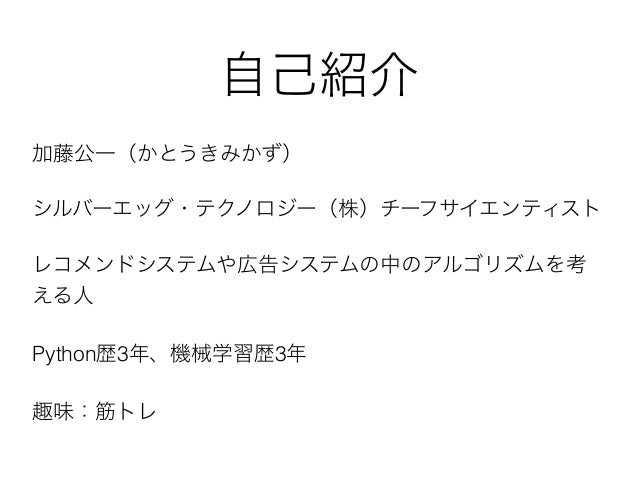23

Share

# Pythonで機械学習入門以前

2016/6/7 みんなのPython勉強会で発表した資料です。

scikit-learnの初心者向けに、データのまとめ方やドキュメントを読む時の心構えについて書いてあります。

See all

### Pythonで機械学習入門以前

1. 1. Python 2016/6/7 Python
2. 2. Python 3 3
3. 3. Python http://bit.ly/yoseiml
4. 4. Python • • scikit-learn • Numpy/Scipy •
5. 5. • • • • • •
6. 6. scikit-learn model = SomeAlogrithm(hyperparameters) model.fit(x,y) prediction = model.predict(z) model = SomeAlogrithm(hyperparameters) model.fit(x) prediction_x = model.labels_ prediction_z = model.predict(z) model = SomeAlogrithm(hyperparameters) model.fit(x) transformed = model.transform(z)
7. 7. scikit-learn n×m n×1 n
8. 8. from sklearn import datasets from sklearn.svm import SVC iris=datasets.load_iris() data_train=iris.data[:-10,:] target_train=iris.target[:-10] data_eval=iris.data[-10:,:] target_eval=iris.target[-10:] svc=SVC() svc.fit(data_train,target_train) predicted=svc.predict(data_eval) print("Accuracy: {}".format((target_eval==predicted).sum()/10.))
9. 9. scikit-learn • • scikit-learn • • •
10. 10. • • •
11. 11.
12. 12. 0 1 … 0 1 … 1 /1 Python i j (i,j)
13. 13. 0 1 2 3 4 5 6 7 8 9 10 11 a 1 [3,4,5] 0 [0,3,6,9] (2,1) a[2,1] 1 a[1,:] 0 a[:,0] (2,1) 7 >>> import numpy as np >>> a=np.arange(12).reshape(4,3) >>> a array([[ 0, 1, 2], [ 3, 4, 5], [ 6, 7, 8], [ 9, 10, 11]]) >>> a[1,:] array([3, 4, 5]) >>> a[2,1] 7 >>> a[:,0] array([0, 3, 6, 9]) >>>
14. 14. csv 9 10 import numpy as np import csv data = [] target = [] filename = "input_data.csv" with open(filename) as f: for row in csv.reader(f): data.append([float(x) for x in row[:9]]) target.append(float(row)) data = np.array(data) target = np.array(target)
15. 15. • • • np.array
16. 16. MovieLens from scipy import sparse items = [] users = [] ratings = [] for line in open("ml-100k/u.data"): a = line.split("t") users.append(int(a)) items.append(int(a)) ratings.append(int(a)) n_users = max(users) n_items = max(items) mat = sparse.lil_matrix((n_users, n_items)) for u, i, r in zip(users, items, ratings): mat[u - 1, i - 1] = r mat = mat.tocsr()
17. 17. • lil_matrix • csr_matrix
18. 18. scikit-learn
19. 19. • • • • •
20. 20. scikit-learn …
21. 21. • • SVM SVC • • SVM • •
22. 22. scikit-learn
23. 23. np.meshgrid? np.c_? ravel?? ???
24. 24. … model = SomeAlogrithm(hyperparameters) model.fit(x,y) prediction = model.predict(z)
25. 25. • scikit-learn • • scikit-learn numpy matplotlib
26. 26.
27. 27.
28. 28. Python http://bit.ly/yoseiml
29. 29. scikit-learn • • • • OK
•#### hiroakiusui3

Jul. 9, 2019
•#### TakahiroOhori1

Jun. 13, 2019
•#### ShusukeOkita

May. 28, 2019
•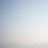#### yasuhirosakuramoto

Jul. 5, 2017
•#### RyousukeNakazato

Jul. 5, 2017
•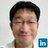#### ssuserd807c4

Jul. 4, 2017
•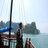#### atsushimori0521

Nov. 9, 2016
•#### MasahiroTabata

Nov. 9, 2016
•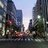#### yuukiebihara79

Jul. 9, 2016
•#### hiroyukikomatsuzawa

Jul. 7, 2016
•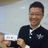#### mackenichi

Jun. 21, 2016
•Jun. 11, 2016
•#### YutakaMiyaji

Jun. 9, 2016
•#### DaikiTsukahara1

Jun. 9, 2016
•#### 36ra3

Jun. 9, 2016
•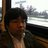#### chroum

Jun. 9, 2016
•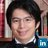Jun. 9, 2016
•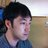#### dyagi1983

Jun. 9, 2016
•Jun. 9, 2016
•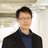Jun. 8, 2016

Total views

35,606

On Slideshare

0

From embeds

0

Number of embeds

20,059

53

Shares

0